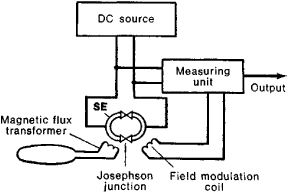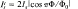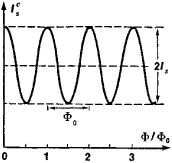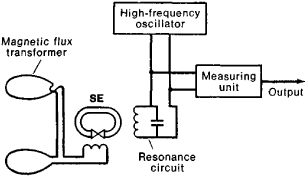Superconducting Magnetometer

The following article is from The Great Soviet Encyclopedia (1979). It might be outdated or ideologically biased.

Superconducting Magnetometer

a quantum magnetometer whose operation is based on the Josephson effect. The sensitivity of such magnetometers reaches 10-9 gauss (G), or 10-13 tesla (T). For measurements of the gradient of a magnetic field, the sensitivity is ~10-10 G/cm, or 10-12 T/m. The sensing element is a superconducting loop containing Josephson junctions, which divide the superconductor. Such a junction can consist of a thin insulating film, approximately 10 angstroms in thickness, or of a point contact. The sensing element reacts to a change in the strength (induction) of a magnetic field threading the superconducting loop.

Figure 1 gives a schematic of a superconducting magnetometer that contains two identical Josephson junctions connectedFigure 1. Schematic of a superconducting magnetometer used to measure the strength (induction) of a magnetic field; the magnetometer has two Josephson junctions connected in parallel: (SE) sensing element

in parallel to a source of direct current. The current Ics that destroys the superconductivity of the sensing element depends on the electrical characteristics of the junctions and on the magnitude Φ of the magnetic flux threading the loop:Here, Is is the critical current that destroys the superconductivity of each of the contacts, and Φ0 = 2 X 10-7 G-cm2 is the magnetic flux quantum—the magnetic flux through the superconducting loop is quantized (see). The critical current should be small: Is ~ Φ0/L, where L is the inductance of the loop. As the flux Φ changes, the current Ics in the loop undergoes oscillations (Figure 2). The current reaches its maximum value each time the changing flux Φ is equal to an integral number of flux quanta Φ0. In other words, the oscillation is equal to a quantum of magnetic flux. When a direct current ~lcs passes through the sensing element, the voltage across the junction also varies periodically with Φ. The flux Φ can be determined from the number of oscillations. Thus, if the area S of the superconducting loop is known, the strength H of the magnetic field under investigation can be found: H = Φ/S.Figure 2. Oscillations of the current flowing in a superconducting loop with two Joseph-son junctions connected in parallel

In order to improve the operational reliability of a superconducting magnetometer, a periodic modulating magnetic field is usually excited in the loop. The excited alternating field has an amplitude ≤Φ0/2S. When such a modulating field is present, there appears in the loop an alternating voltage whose phase changes in direct proportion to the external field H. The measuring unit of the magnetometer amplifies the alternating component of the loop voltage and discriminates the phase change. The signal obtained at the output of the measuring unit is proportional to the phase change and, consequently, to the value of H.

Superconducting magnetometers are also made that have a source (oscillator) of alternating current of frequency 107-109 hertz (Hz) and a single Josephson junction in the sensing element (Figure 3). Current is induced in the sensing element by means of a resonant circuit tuned to the oscillator frequency. At the same time, a low-frequency (~103 Hz) alternating current, which is flowing through the same circuit, modulates the magnetic field in the sensing element. The volt-ampere characteristic of the sensing element is nonlinear with respect to the magnetic field passing through the resonant circuit. For this reason, the phase of the low-frequency modulation varies as a function of the magnitude of the external magnetic field, that is, the field under investigation. The external magnetic field is supplied to the sensing element by means of a magnetic field transformer, which consists of a pickup loop and a coil that is inductively coupled with the sensing element. Superconducting wire is used for the transformer winding, and the flux is transferred without losses. In this type of superconducting magnetometer, the transformer has two input loops connected in opposition. When the loops are connected in this way, the sensing element responds to the gradient of the field, and is thus a gradiometer. The magnetometer’s measuring unit amplifies the modulated high-frequency signal and detects it. As a result, the low-frequency signal is discriminated whose phase is proportional to the field gradient being measured.

The very high sensitivity of superconducting magnetometers permits a number of delicate experiments to be carried out with such devices. For example, the values of physical constants can be refined, and voltages can be measured that are as small as 10~14 volt. Other uses include the making of magnetocardio-grams.Figure 3. Schematic of a superconducting gradiometer, that is, a magnetometer used to measure the gradient of a magnetic field: (SE) sensing element

REFERENCES

Feynman, R. R. Leighton, and M. Sands. Feinmanovskie lektsii po fizike, vol. 9. Moscow, 1967. (Translated from English.)
Clarke, J. “Nizkochastotnye primeneniia sverkhprovodiashchikh kvantovykh interferentsionnykh ustroistv.” Tr. In-ta inzhenerov po elektronike i radioelektronike, 1973, vol. 61, no. 1, p. 9.
Zavaritskii, N. V., and A. N. Vetchinkin. “Ustanovka SKIMP.” Pribory i tekhnika eksperimenta, 1974, no. 1.

N. V. ZAVARITSKII